# Tree Tutorial 5: Identity Rules

Logical System

### Skills to be acquired in this tutorial:

To become familiar with the new rules for predicate logic trees with identity.

### Tutorial:

It is possible to use trees with formulas containing identity. Really there are two different ways to implement it: either there are two new rules, or there is one new rule and a new way of closing a branch from a single formula. Both will be described.

With identity, at a rough and hand-waving level, two different things might go on. First, everything is identical to itself ie a=a, b=b, c=c, etc., in sum ∀x(x=x). Then, identities are used to substitute for one term in another, so, for example, Fa and a=b allow the inference to Fb. Building these into tree rules is awkward in both cases.

#### The two new rules approach

To cover everything being identical with itself, there is an Identity Introduction rule which grows a tree by adding ∀x(x=x) (in turn, this is almost always followed by Universal Decomposition, the tree counterpart of Universal Instantiation, to get a=a or m=m or a formula saying that a term is identical to itself, for some desired term).

This Identity Introduction is slightly different to the rule Howson Logic with Trees uses. Howson omits adding the ∀x(x=x) step and allows you to go to a=a, etc. . This difference is of no great consequence. The Howson may be quicker and better when using pencil on paper, but the software in use here has to find out from the User certain information (on the desired term to use in the identity) and the 'extra' step is just one transparent, and logically sound, way of doing this.

Identity Introduction is at stress with the general tree approach which is decompositional ie it takes things apart. To use Identity Introduction, a formula is selected and then 'Identity Introduction' from the menu. From a logical point of view, there is no need to select a formula. However, when extending a tree, the User may have several open branches, and wish to have the identity in one branch and not the others-- selecting a formula picks that branch. Here are 3 examples of Indentity Introduction in use. The (valid) example argument is

a=b∴ (a=a&b=a)

and the tree for it starts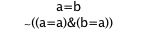now, Identity Introduction could be used immediately to give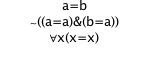or the ~.D tree rule could have been used first, and then Identity Introduction used on the left branch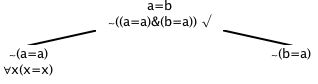or on the right branch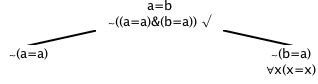[We will see which of these are useful, shortly.]

The second identity rule is the one that allows substitutions. It also is unusual in that it requires two formulas before it can be used (all the other tree rules decompose one formula, apart from Identity Introduction, which extends from nothing). One of these two formulas needs to be an identity statement, and the other can be any statement containing one of the terms. Here is a suitable tree, with the two formulas selected. [To select two formulas, you click on one, then click on the second.]and what the rule allows you to do is to extend the tree by substituting for one or more of the terms in the 'any' statement. So, here is one growthand anotherand another(which you do depends on the purposes at hand).

Now we can show how to close that first tree (for the valid argument a=b∴ (a=a&b=a))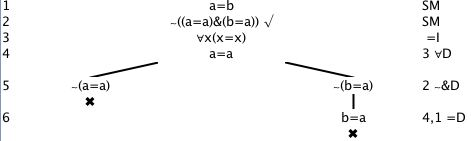You may have wondered where line 6, the b=a, came from. The display has been expanded out to show you—it comes from lines 1 and 4 and substituting for the first a in line 4.

There is a restriction to the substitution rule. Were the identity to contain variables, there could be problems with 'capturing' when a substitution is made. So, the terms in the identity must be variable free. So, an identity like a=b is fine, because both the terms are constants, so too is f(a)=h(c) because these complex terms do not contain any variables. On the other hand, a=x or f(x)= h cannot be used, because some of the terms contain variables. There is a label to describe terms of the desired kind. A closed term is either a constant or a complex (compound) term whose subterms are themselves closed terms. Then for the tree Identity Decomposition rule, the terms in the identity have to be closed terms.

#### The one new rule approach (and a shortcut on closing branches)

Usually when we close a branch we require the selection of two formulas, one of which being the negation of the other. When identities are in use occasionally you will come across a formula like ¬(a=a) . Now, this obviously cannot be true or satisfied, no matter what. And we could close a branch containing it, under the two rule approach to identity, by creating the additional formula (a=a) by Identity Introduction and selecting both those lines and closing the branch. But we allow a shortcut. If there is a formula which says that something is not identical to itself, eg ~(a=a),~(b=b), ~(f(t)=f(t)), etc. just select that one formula alone and you can close the branch off the Actions menu.

It turns out that if you have this shortcut, ie the ability to close a branch from a single formula of the form ~(a=a), you do not need the Identity Introduction rule at all.  All the closings that need to be done using identity can be done with just the substitution rule.

#### The software implementation

The software implements both rules and closure of a branch from a single suitable line. What you use is up to you. You need never use the Identity Introduction rule, provided you are willing to close from a single formula denial of self identity. You need never close from a single formula denial of identity, providing you are willing to use both Identity rules (as and when required).

There are two other implementation issues with Identity and trees. Some implementations require that the formula being substituted into be a 'literal' , that is either an atomic formula or the negation of an atomic formula. With this kind of implementation, with the formulas

a=b
Fa
~Fa
Fa &~Fa

the identity can be used to substitute into the second and third formulas ie Fa, ~Fa (they are literals) but not the fourth formula Fa & ~Fa (because it is not a literal). [You'd have to split the fourth one up, to get literals, and then you can substitute.] The software here allows substitution into any suitable formula, that formula does not have to be a literal.

Then, 'free variables' raise questions. If the identity is something like

a=x

where x is a free variable, should substitutions be permitted in other formulas? Probably the answer is that it is alright (but there may be other qualifications that come in). However a formula like

a=x

is an oddity. What is really says or means is

∀x(a=x)

and from this a=a, a=b, a=c, etc all follow (all of which can be used in substitutions). Not only is a formula like a=x an oddity, it is rare (it is not easy to get it naturally in a branch of a tree). We will just pass on free variables.

The software just rules free variables out. The terms in the identity have to be 'closed terms'.

## Exercises to accompany Tree Tutorial 5

### Exercise 1 (of 2):

Determine whether these arguments are valid (ie try to produce closed trees for them). [These three statements are known as the axioms for identity (reflexivity, symmetry, and transitivity).]

a) ∴∀x(x=x)
b) ∴∀x∀y(x=y →y=x)
c) ∴∀x∀y∀z((x=y&y=z) →y=z)

### Exercise 2 (of 2):

Determine whether these arguments are valid (ie try to produce closed trees for them)

d) ∀xF(x,x),∃x∃y~F(x,y) ∴ ∃x~(x=a)
e) ∀x(F(x,c)→x=a), ~c=a ∴ ∀x(~x=c)
f) ∀x(x=a→G(x,b)), ~∃xG(x,x)∴ ~a=b

If you decide to use the web application for the exercises you can launch it from here Deriver [default] — username 'logic' password 'logic'. Then either copy and paste the above formulas into the Journal or use the Deriver File Menu to Open Web Page with this address https://softoption.us/test/treesStandAlone/CombinedTutorialsDefault.html . Then select the desired formula(s) and Start Tree off the Actions Menu.

Preferences

You will need to set some Preferences for this. Set identity to true (and that will give you the identity rules); and  you can check that the parser is set to default.